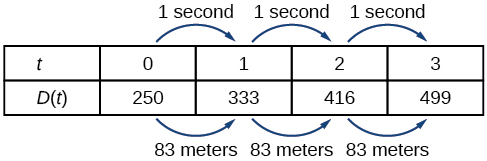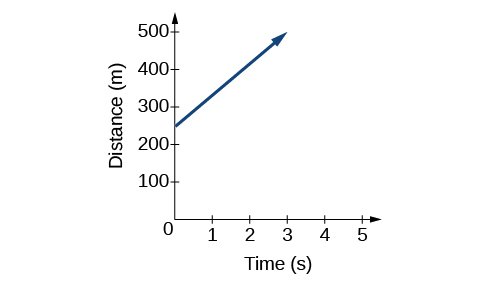# 2.1 Linear functions  (Page 2/17)

 Page 2 / 17
$D\left(t\right)=83t+250$

## Representing a linear function in tabular form

A third method of representing a linear function is through the use of a table. The relationship between the distance from the station and the time is represented in [link] . From the table, we can see that the distance changes by 83 meters for every 1 second increase in time.Tabular representation of the function D showing selected input and output values

Can the input in the previous example be any real number?

No. The input represents time, so while nonnegative rational and irrational numbers are possible, negative real numbers are not possible for this example. The input consists of non-negative real numbers.

## Representing a linear function in graphical form

Another way to represent linear functions is visually, using a graph. We can use the function relationship from above, $D\left(t\right)=83t+250,$ to draw a graph, represented in [link] . Notice the graph is a line. When we plot a linear function, the graph is always a line.

The rate of change, which is constant, determines the slant, or slope of the line. The point at which the input value is zero is the vertical intercept, or y -intercept , of the line. We can see from the graph in [link] that the y -intercept in the train example we just saw is $\left(0,250\right)$ and represents the distance of the train from the station when it began moving at a constant speed.The graph of D ( t ) = 83 t + 250. Graphs of linear functions are lines because the rate of change is constant.

Notice that the graph of the train example is restricted, but this is not always the case. Consider the graph of the line $f\left(x\right)=2{x}_{}+1.$ Ask yourself what numbers can be input to the function, that is, what is the domain of the function? The domain is comprised of all real numbers because any number may be doubled, and then have one added to the product.

## Linear function

A linear function    is a function whose graph is a line. Linear functions can be written in the slope-intercept form of a line

$f\left(x\right)=mx+b$

where $b$ is the initial or starting value of the function (when input, $x=0$ ), and $m$ is the constant rate of change, or slope    of the function. The y -intercept is at $\left(0,b\right).$

## Using a linear function to find the pressure on a diver

The pressure, $P,$ in pounds per square inch (PSI) on the diver in [link] depends upon her depth below the water surface, $d,$ in feet. This relationship may be modeled by the equation, $P\left(d\right)=0.434d+14.696.$ Restate this function in words.

To restate the function in words, we need to describe each part of the equation. The pressure as a function of depth equals four hundred thirty-four thousandths times depth plus fourteen and six hundred ninety-six thousandths.

## Determining whether a linear function is increasing, decreasing, or constant

The linear functions we used in the two previous examples increased over time, but not every linear function does. A linear function may be increasing, decreasing, or constant. For an increasing function    , as with the train example, the output values increase as the input values increase. The graph of an increasing function has a positive slope. A line with a positive slope slants upward from left to right as in [link] (a) . For a decreasing function    , the slope is negative. The output values decrease as the input values increase. A line with a negative slope slants downward from left to right as in [link] (b) . If the function is constant, the output values are the same for all input values so the slope is zero. A line with a slope of zero is horizontal as in [link] (c) .

a colony of bacteria is growing exponentially doubling in size every 100 minutes. how much minutes will it take for the colony of bacteria to triple in size
what is the importance knowing the graph of circular functions?
can get some help basic precalculus
What do you need help with?
Andrew
how to convert general to standard form with not perfect trinomial
can get some help inverse function
ismail
Rectangle coordinate
how to find for x
it depends on the equation
Robert
whats a domain
The domain of a function is the set of all input on which the function is defined. For example all real numbers are the Domain of any Polynomial function.
Spiro
foci (–7,–17) and (–7,17), the absolute value of the differenceof the distances of any point from the foci is 24.
difference between calculus and pre calculus?
give me an example of a problem so that I can practice answering
x³+y³+z³=42
Robert
dont forget the cube in each variable ;)
Robert
of she solves that, well ... then she has a lot of computational force under her command ....
Walter
what is a function?
I want to learn about the law of exponent
explain this
what is functions?
A mathematical relation such that every input has only one out.
Spiro
yes..it is a relationo of orders pairs of sets one or more input that leads to a exactly one output.
Mubita
Is a rule that assigns to each element X in a set A exactly one element, called F(x), in a set B.
RichieRich
If the plane intersects the cone (either above or below) horizontally, what figure will be created?ByByBy Brooke DelaneyBy OpenStaxByBy OpenStaxBy Madison ChristianBy Anonymous UserBy Keyaira BraxtonBy Joanna SmithbackBy OpenStaxBy OpenStax